• hslogic算法仿真-通过MATLAB实现图像库人脸剪切、旋转，通过剪切，旋转，得到标准的人脸区域
• Matlab空间变换函数 imtransform 可以实现图像仿射变换(如 平移、旋转剪切、缩放)、投影变换, 该函数可与 maketform 配合使用。不过官网目前更推荐使用 imwarp 用法说明： B = imtransform(A,tform) 根据 tform ...
Matlab空间变换函数 imtransform
Matlab空间变换函数 imtransform 可以实现图像仿射变换(如 平移、旋转、剪切、缩放)、投影变换, 该函数可与 maketform  配合使用。不过官网目前更推荐使用 imwarp
用法说明：

B = imtransform(A,tform)
根据 tform 定义的二维空间变换来变换图像 A，并返回变换后的图像 B。如果 A 是彩色图像，则 imtransform 会对每个颜色通道应用相同的二维变换。类似地，如果 A 是三维体或具有三个或更多维度的图像序列，则 imtransform 将相同的二维变换应用于沿更高维度的所有二维平面。对于任意维度的数组变换，请使用 tformarray。
B = imtransform(A,tform,interp)
指定要使用的插值形式。
B = imtransform(___,Name,Value)
使用名称-值对组来控制空间变换的各个方面。
[B,xdata,ydata] = imtransform(___)
还返回输出图像 B 在输出 X-Y 空间中的范围。默认情况下，imtransform 会自动计算 xdata 和 ydata，使得 B 包含整个变换后的图像 A。但是，您可以通过为 XData 和 YData 名称-值对组输入参数指定值来覆盖此自动计算。

变换的原理： (x’, y’) = T(x, y)
x' = a0x + a1y + a2
y' = b0x + b1y + b2
矩阵形式：我们通过控制 a0, a1, a2, b0, b1, b0 来达到图像变换的目的
以下表格为具体变换数值：

放射性变换类型
a0
a1
a2
b0
b1
b0

平移Δx, Δy
1
0
Δx
0
1
Δy

缩放[Sx, Sy]
Sx
0
0
0
Sy
0

逆时针旋转角度
cosθ
sinθ
0
-sinθ
cosθ
0

水平切变shx
1
0
0
shx
1
0

垂直切变shy
1
shy
0
0
1
0

整体切变[shx, shy]
1
shy
0
shx
1
0

以下代码演示图像旋转、缩放、平移、整体切变：
I = imread('火影2.jpg');

%实现图像旋转
Ia = maketform('affine', [cosd(30) -sind(30) 0; sind(30) cosd(30) 0; 0 0 1]);
Ia = imtransform(I, Ia);

%实现图像缩放
Ib = maketform('affine', [5 0 0; 0 10.5 0; 0 0 1]);
Ib = imtransform(I, Ib);

%实现图像平移
xform = [1 0 55; 0 1 115; 0 0 1]';
Ic = maketform('affine', xform);
Ic = imtransform(I, Ic, 'XData', ...
[1 (size(I, 2) + xform(3, 1))], 'YData', ...
[1 (size(I, 1) + xform(3, 2))], 'FillValues', 255);

%实现图像整体切变
Id = maketform('affine', [1 4 0; 2 1 0; 0 0 1]);
Id = imtransform(I, Id, 'FillValues', 255);

figure;
subplot(231);imshow(I);	 %显示原图像
subplot(232);imshow(Ia); %旋转
subplot(233);imshow(Ib); %缩放
subplot(234);imshow(Ic); %平移
subplot(235);imshow(Id); %整体切变

结果演示：完整目录
Matlab数字图像处理——图像处理工具箱Image Processing Toolbox
Matlab数字图像处理——图像类型的转换
Matlab数字图像处理——图像文件的读取
Matlab数字图像处理——图像文件的显示
Matlab数字图像处理——视频文件的读写
Matlab数字图像处理——图像的像素运算(灰度变换)
Matlab数字图像处理——图像的空间变换
Matlab数字图像处理——图像的平移、邻域操作、区域选取
Matlab数字图像处理——图像增强
Matlab数字图像处理——图像复原


展开全文• 空间变换 函数：intransform() 仿射变换（平移、缩放、旋转剪切）、投影变换 （x’,y’）=T(x,y) x’=a0x+a1y+a2 y’=b0x+b1y+b2
空间变换
函数：intransform()
仿射变换（平移、缩放、旋转、剪切）、投影变换
（x’,y’）=T(x,y)
x’=a0x+a1y+a2
y’=b0x+b1y+b2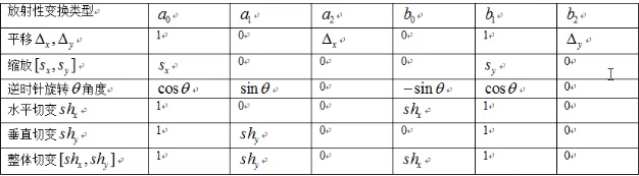maketform、intransform
close all;
clear all;
clc;
[I,map]=imread("E:\Matlab_exercise\图片素材\lian2.jpg");
Ia=maketform("affine", ...
[cosd(30) -sind(30) 0; sind(30) cosd(30) 0; 0 0 1]');%创建旋转参数结构体
Ia=imtransform(I,Ia,'FillValues',255);%实现图像缩放

Ib=maketform("affine",[5 0 0;0 10.5 0;0 0 1]');%创建缩放参数结构体
Ib=imtransform(I,Ib);%实现图像旋转

xform=[1 0 55;0 1 115;0 0 1]';%创建图像平移参数结构体
Ic=maketform("affine",xform);
Ic=imtransform(I,Ic,'XData',...%实现图像平移
[1 (size(I,2)+xform(3,1))],'YData',...
[1 (size(I,1)+xform(3,2))],'FillValues',255);

Id=maketform("affine",[1 4 0;2 1 0;0 0 1]');%创建图像整体切变的参数结构体
Id=imtransform(I,Id,'FillValues',255);

set(0,'defaultFigurePosition',[100,100,1000,500]);
set(0,'defaultFigureColor',[1 1 1]);

figure;
imshow(I);

figure;
subplot(121),imshow(Ia),axis on;
subplot(122),imshow(Ib),axis on;

figure;
subplot(121),imshow(Ic),axis on;
subplot(122),imshow(Id),axis on;

图像平移
平移函数
function J = move(I,a,b)
%I为原图像,a,b为沿X,Y轴移动的距离
[M,N,G]=size(I);
I=im2double(I);
J=ones(M,N,G);
for i=1:M
for j=1:N
if((i+a)>=1&&(i+a)<=M&&(j+b)>=1&&(j+b)<=N)%判断平移后行列坐标是否超出范围
J(i+a,j+b,:)=I(i,j,:);%进行图像平移
end
end
end
end

close all;clear all;clc;
I=imread('E:\Matlab_exercise\图片素材\beauty.jpg');
a=50;b=50;
J1=move(I,a,b);

a=-50;b=50;
J2=move(I,a,b);

a=50;b=-50;
J3=move(I,a,b);

a=-50;b=-50;
J4=move(I,a,b);

set(0,'defaultFigurePosition',[100,100,1000,500]);
set(0,'defaultFigureColor',[1 1 1]);

figure;
imshow(I);
figure;
subplot(121),imshow(J1),axis on;
subplot(122),imshow(J2),axis on;
figure;
subplot(121),imshow(J3),axis on;
subplot(122),imshow(J4),axis on;



展开全文• 图像的几何变换 图像的几何变换是将一幅图像中的坐标映射到另外一幅图像中的新坐标位置，它不改变图像的像素值，只是改变像素所在的几何位置，使原始图像按照...5、图像旋转 6、图像剪切 7、图像的空间变换 ...
图像的几何变换
图像的几何变换是将一幅图像中的坐标映射到另外一幅图像中的新坐标位置，它不改变图像的像素值，只是改变像素所在的几何位置，使原始图像按照需要产生位置、形状和大小的变换。
1、图像的平移
在MATLAB中，没有提供具体图像平移函数，直接运用MATLAB指令编程即可实现图像的平移操作。
MATLAB实现
示例：
构造图像平移函数
function [output_image] = move(input_image,m,n)
%定义move函数，input_image为输入图像，m为输入图像沿着水平方向移动的距离，n为输入图像沿着垂直方向移动的距离，output_image为平移后的输出图像
%不考虑图像平移以后的溢出情况，找不到对应点的地方都赋值为1
[M,N,G]=size(input_image);    %获取输入图像的大小
double_image=im2double(input_image);   %将数据图像类型转换成双精度
new_image=ones(M,N,G);    %初始化新图像矩阵全为1，大小与输入图像相同
for i=1:M
for j=1:N
if((i+m)>=1&&(i+m)<=M&&(j+n)>=1&&(j+n)<=N)      %判断平移以后行列坐标是否超出范围
new_image(i+m,j+n,:)=double_image(i,j,:);    %进行图像平移
end
end
end
output_image=new_image;

调用函数，实现图像平移
clear all; close all; clc;
input=imread('G:\picture\Fig0450(a)(woman_original).tif');
output1=move(input,100,100);
output2=move(input,-100,-100);
subplot(1,3,1); imshow(input); title('原图像');
subplot(1,3,2); imshow(output1); title('右下平移后的图像');
subplot(1,3,3); imshow(output2); title('左上平移后的图像');

运行结果：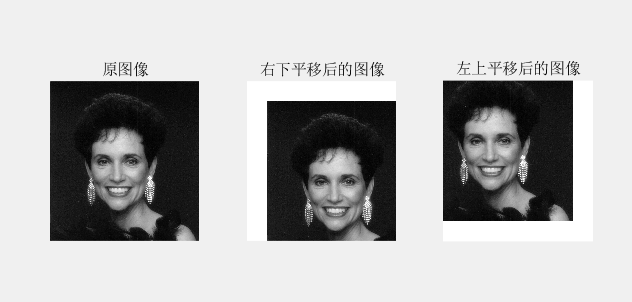2、图像的镜像
图像的镜像分为垂直镜像和水平镜像。
MATLAB实现
示例：
构造图像镜像函数
function [outImage] = mirror(InImage,n)
%定义mirror函数实现图像镜像变换
%参数n为1时，实现水平镜像变换
%参数n为2时，实现垂直镜像变换
%参数n为3时，实现水平垂直镜像变换
[M,N,G]=size(InImage); %获取输入图像InImage的大小
transImage=InImage;
if n==1
for i=1:M
for j=1:N
transImage(i,M+1-j,:)=InImage(i,j,:);
end
end
elseif n==2
for i=1:M
for j=1:N
transImage(M+1-i,j,:)=InImage(i,j,:);
end
end
elseif n==3
for i=1:M
for j=1:N
transImage(M+1-i,M+1-j,:)=InImage(i,j,:);
end
end
else
error('参数n输入不正确，n取1、2、3');
end
outImage=transImage;

调用函数，实现图像镜像
clear all; close all; clc;
input=imread('G:\picture\Fig0450(a)(woman_original).tif');
output1=mirror(input,1);
output2=mirror(input,2);
output3=mirror(input,3);
subplot(2,2,1); imshow(input); title('原图像');
subplot(2,2,2); imshow(output1); title('水平镜像');
subplot(2,2,3); imshow(output2); title('垂直镜像');
subplot(2,2,4); imshow(output3); title('水平垂直镜像');

运行结果：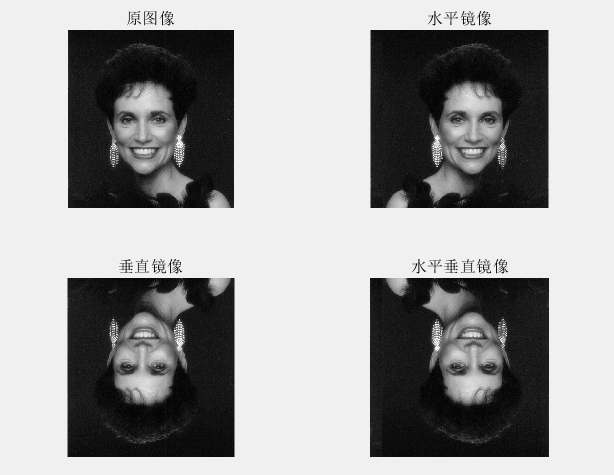3、图像的缩放
图像的缩放是指将给定的图像在x轴方向按比例缩放fx倍，在y轴方向按比例缩放fy倍，从而获得一幅新的图像。如果fx=fy，即在x轴方向和y轴方向缩放的比例相同，即为图像的全比例缩放。如果fx≠fy，图像比例缩放会改变原始图像像素间的相对位置，产生几何畸变。
–imresize()函数
MATLAB实现
示例：
clear all; close all; clc;
InImage=imread('G:\picture\Fig0450(a)(woman_original).tif');
shrink1=imresize(InImage,0.5);  %设置缩放比例，缩小图像
enlarge1=imresize(InImage,2);  %设置缩放比例，扩大图像
shrink2=imresize(InImage,[500 600]);  %设置缩放后的图像行列，实现缩放图像并显示
shrink3=imresize(InImage,[NaN 600]);  %函数按照输入图像纵横比生成行数，实现缩放图像并显示
enlarge2=imresize(InImage,1.5,'bilinear');  %采用双线性插值法对图像进行缩放
enlarge3=imresize(InImage,1.5,'triangle');  %采用三角型核函数插值对图像进行缩放

subplot(2,4,1); imshow(InImage); title('原图像');
subplot(2,4,2); imshow(shrink1); title('shrink1');
subplot(2,4,3); imshow(enlarge1); title('enlarge1');
subplot(2,4,4); imshow(shrink2); title('shrink2');
subplot(2,4,5); imshow(shrink3); title('shrink3');
subplot(2,4,6); imshow(enlarge2); title('enlarge2');
subplot(2,4,7); imshow(enlarge3); title('enlarge3');

运算结果：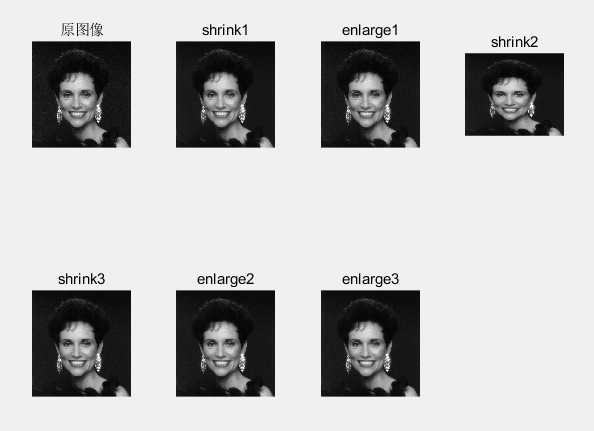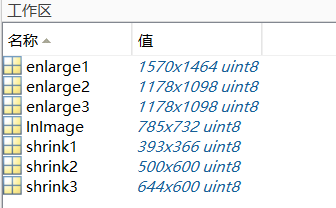4、图像的转置
图像转置即为图像的行列坐标互换，进行图像转置后，图像的大小会发生变化。
MATLAB实现
示例：
function [outputImage] = transposition(inputImage)
% inputImage为输入图像
% outputImage为对输入图像转置的输出图像
[M,N,G]=size(inputImage);
inputImage=im2double(inputImage);
transp=ones(N,M,G);
for i=1:M
for j=1:N
transp(j,i,:)=inputImage(i,j,:);
end
end
outputImage=transp;
end

运行结果：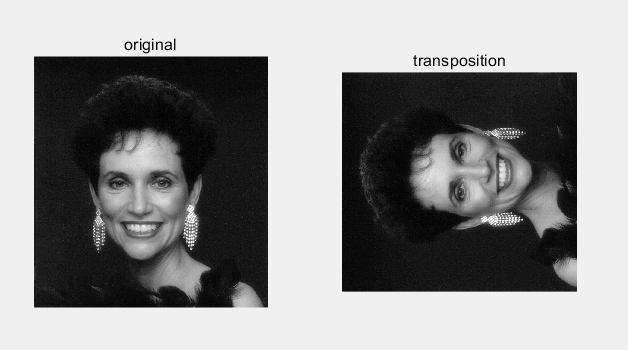5、图像的旋转
图像的旋转变换属于图像的位置变换，通常是以图像的中心为原点，将图像上的所有像素都旋转一个相同的角度。旋转后，图像的大小一般会改变。
–imrotate()函数
MATLAB实现
示例：
clear all; close all; clc;
InImage=imread('G:\picture\Fig0227(a)(washington_infrared).tif');
rotate1=imrotate(InImage,20); %将图像以其中心为原点逆时针旋转20°，采用最近邻插值生成完整旋转图像
rotate2=imrotate(InImage,-20); %将图像以其中心为原点顺时针旋转20°，采用最近邻插值生成完整旋转图像
rotate3=imrotate(InImage,20,'bilinear'); %将图像以其中心为原点逆时针旋转20°，采用双线性插值生成完整旋转图像
rotate4=imrotate(InImage,20,'bilinear','crop'); %将图像以其中心为原点逆时针旋转20°，采用最近邻插值,对旋转后图像裁剪保证输出图像与输入图像大小相等
rotate5=imrotate(InImage,20,'bilinear','loose'); %将图像以其中心为原点逆时针旋转20°，采用最近邻插值生成完整旋转图像

subplot(2,3,1); imshow(InImage);
subplot(2,3,2); imshow(rotate1);
subplot(2,3,3); imshow(rotate2);
subplot(2,3,4); imshow(rotate3);
subplot(2,3,5); imshow(rotate4);
subplot(2,3,6); imshow(rotate5);

运行结果：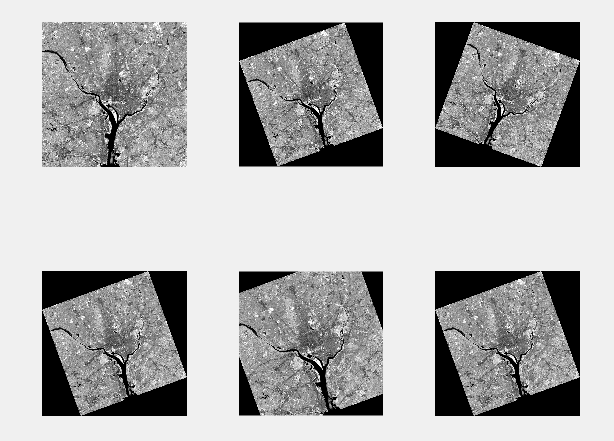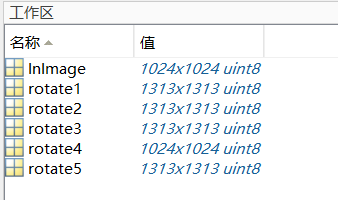6、图像的剪切
–imcrop()函数
MATLAB实现
示例：
clear all; close all; clc;
InImage=imread('G:\picture\Fig0227(a)(washington_infrared).tif');
rect=[445 625 130 200]; %定义剪切区域
X=imcrop(InImage,rect); %进行图像剪切
subplot(1,2,1); imshow(InImage); title('原图像');
rectangle('Position',rect,'LineWidth',2,'EdgeColor','r'); %将图像的剪切区域标出
subplot(1,2,2); imshow(X); title('剪切图像');

运行结果：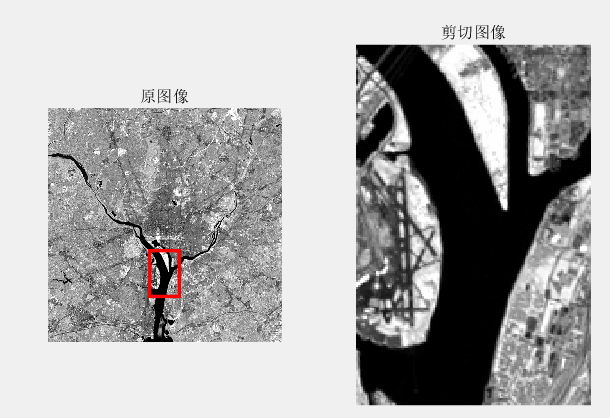7、图像的空间变换
在MATLAB的图像处理工具箱中提供了一个专门的函数 intransform（），可以通过定义参数实现多种类型的空间变换，包括仿射变换（如平移、缩放、旋转、剪切）、投影变换等。


展开全文• 本部分内容主要介绍基于图像像素的运算方法，包括点运算、代数运算、逻辑运算、图像的几何变换方法，包括平移、镜像、缩放、转置、旋转剪切图像的邻域操作和区域选择。 一、图像点运算 1.通过灰度线性变换函数...
本部分内容主要介绍基于图像像素的运算方法，包括点运算、代数运算、逻辑运算、图像的几何变换方法，包括平移、镜像、缩放、转置、旋转及剪切，图像的邻域操作和区域选择。

一、图像点运算

1.通过灰度线性变换函数imadjust()对图像进行线性灰度变换

% 编写时间：2018年3月20日17:45:48
close all; clear all; clc;

gamma = 0.5; % 设定调整线性度取值
I = imread('peppers.png');

% 将图像数据赋值给R，并将原图像变成单色图像，保留红色
R = I;
R(:, :, 2) = 0;
R(:, :, 3) = 0;
% 调整R的灰度，结果返回给R1
R1 = imadjust(R, [0.5 0.8], [0 1], gamma);

% 将图像数据赋值给G，并将原图像变成单色图像，保留绿色
G = I;
G(:, :, 1) = 0;
G(:, :, 3) = 0;
% 调整G的灰度，结果返回给G1
G1 = imadjust(G, [0 0.3], [0 1], gamma);

% 将图像数据赋值给B，并将原图像变成单色图像，保留蓝色
B = I;
B(:, :, 1) = 0;
B(:, :, 2) = 0;
% 调整B的灰度，结果返回给B1
B1 = imadjust(B, [0 0.3], [0 1], gamma);

% 求变换后的RGB图像
I1 = R1 + G1 + B1;

figure('name', 'R');
subplot(121), imshow(R);
subplot(122), imshow(R1);

figure('name', 'G');
subplot(121), imshow(G);
subplot(122), imshow(G1);

figure('name', 'B');
subplot(121), imshow(B);
subplot(122), imshow(B1);

figure('name', 'I');
subplot(121), imshow(I);
subplot(122), imshow(I1);

2.对图像进行分段式灰度变换

% 对图像进行分段式灰度变换
% 编写时间：2018年3月20日20:33:04

close all; clear all; clc;

R = imread('peppers.png');  % 384x512x3 uint8
J = rgb2gray(R); % 将彩色图像数据转换为灰度图像数据
[M, N] = size(J);

for i = 1:M            % 3段线性灰度变换处理
for j = 1:N
if (J(i,j) <= 35);
H(i,j) = J(i,j) * 10;
elseif ( J(i,j)>35 & J(i,j)<=75);
H(i,j) = (10/7) * (J(i,j) - 5) + 50;
else ( J(i,j) > 75 );
H(i,j) = (105/180) * (J(i,j) - 75) + 150;
end
end
end

figure;
subplot(121), imshow(J);
subplot(122), imshow(H);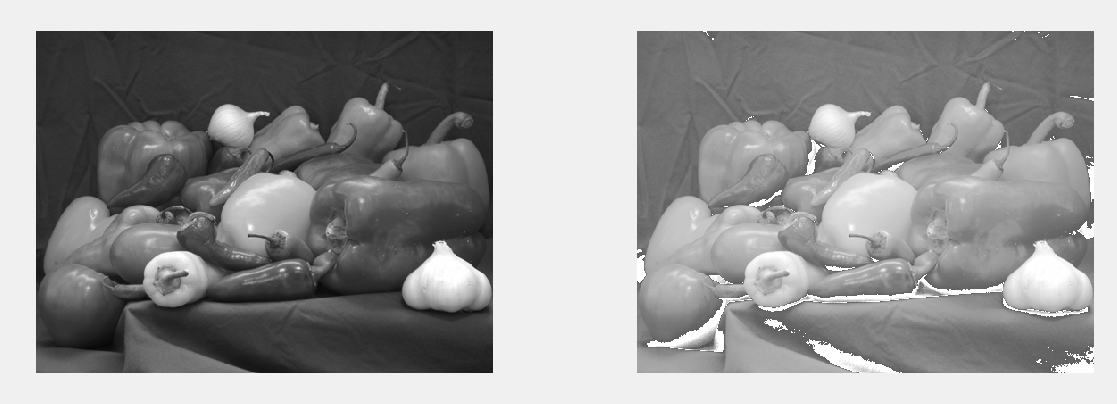3.进行基于常用对数的非线性灰度变换

图像通过对数变换可扩展低值灰度，压缩高值灰度。

% 对图像进行基于常用对数的非线性灰度变换
% 编写时间：2018年3月20日21:25:49

close all; clear all; clc;

R = imread('peppers.png');
G = rgb2gray(R);
J = double(G);
H = (log(J+1)) / 10;

figure;
subplot(131), imshow(R);
subplot(132), imshow(G);
subplot(133), imshow(H);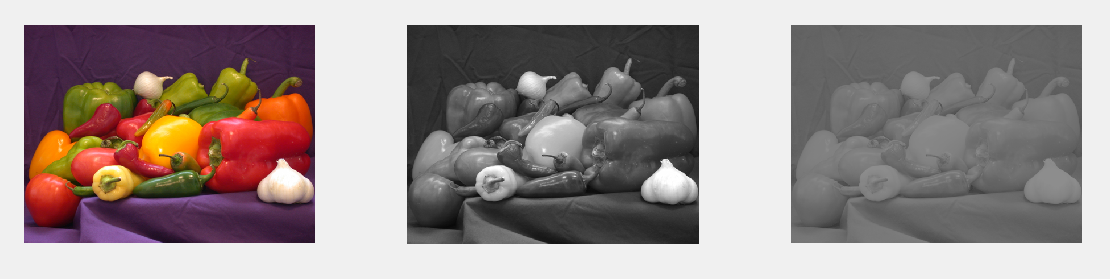二、图像代数运算

图像的代数运算是指将两幅或多幅图像通过对应像素之间的加、减、乘、除运算得到输出图像的方法。在MATLAB中图像数据类型是uint8，当进行代数运算时有可能产生溢出，所以应当在进行图像代数运算之前首先将数据类型转换成double型，从而保证结果的准确性。

1.图像的加法运算

在MATLAB图像处理工具箱中提供的函数imadd()可实现两幅图像的相加或者一幅图像和常数的相加。

1.1 使用加法运算将两幅图像叠加在一起

% 编写时间：2018年3月20日22:07:03
close all; clear all; clc;
% 要求两幅图像的大小相同
I = imread('rice.png');
J = imread('cameraman.tif');
K = imadd(I, J);
figure;
subplot(131), imshow(I);
subplot(132), imshow(J);
subplot(133), imshow(K);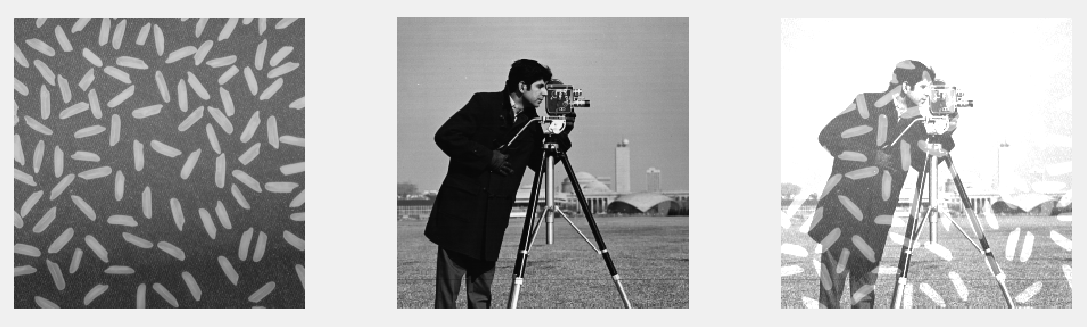1.2 给图像每个像素加一个常数改变图像亮度

% 编写时间：2018年3月20日22:07:03
close all; clear all; clc;
I = imread('rice.png');
J = imadd(I, 30);

figure;
subplot(121), imshow(I);
subplot(122), imshow(J);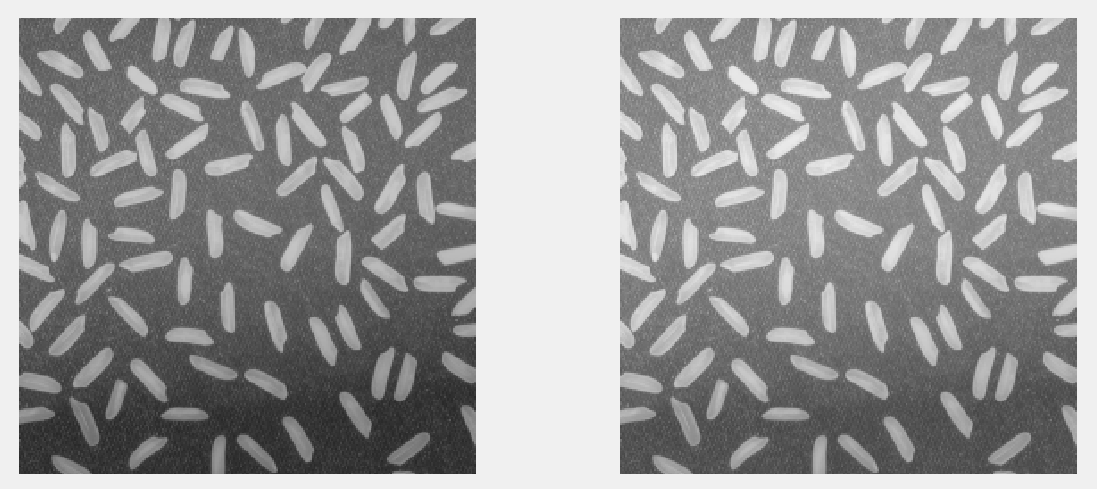1.3使用加法运算消除一幅图像的附加噪声

图像加法运算的另一重要应用是通过同一幅图像叠加取平均，消除原图像中的附加噪声。在MATLAB图像处理工具箱中提供了函数imnoise()实现在图像中加入噪声，其调用格式如下：

J=imnoise(I,type,parameters):该函数是对图像I 添加典型噪声后生成有躁图像，结果返回给J。其中I为原始图像；type为添加的噪声类型，取值可以是高斯噪声gaussian、零均值的高斯噪声localvar、泊松噪声poisson、椒盐噪声salt & pepper和乘性噪声speckle；parameters是不同类型噪声的参数。

% 使用加法运算消除一幅图像的附加噪声
% 编写时间：2018年3月20日22:32:00
close all; clear all; clc;

R = im2double(imread('eight.tif'));
A = imnoise(R, 'gaussian', 0, 0.05); % 均值为0，方差为0.05的高斯噪声

I = A;

M = 3;
for i = 1:M
I = imadd(I, R);
end

avg = I / (M+1); % 这么做只是稀释了高斯噪声

% A2 = imnoise(R, 'gaussian', 0, 0.05);
% A3 = imnoise(R, 'gaussian', 0, 0.05);
% A4 = imnoise(R, 'gaussian', 0, 0.05);
%
% avg = (R+A+A2+A3+A4) / 5;

figure;
subplot(131), imshow(R);
subplot(132), imshow(A);
subplot(133), imshow(avg);

上面代码中的M取值为3，如果需要M可以取任意值，可以将上述代码改写成函数的形式。

function [J, runningtime] = denoise(I, M)
% DENOISE  通过叠加图像消除高斯噪声
% 输入：
%     I -- 原图像
%     M -- 叠加噪声的次数
% 输出：
%     J -- 消除噪声的图像
%     runningtime -- 函数运行的时间

% created by gq 2018年4月19日00:02:58

I = im2double(I);
A = imnoise(I, 'gaussian', 0, 0.05);
temp = A;

tstart = tic; % 开始计时
for i = 1 : M
temp = imadd(temp, I);
end
J = temp / (M+1);
runningtime = toc(tstart); % 计时结束

调用上面的函数：

close all; clear all; clc;

I = imread('eight.tif');
M1 = 3;
[J1, runningtime1] = denoise(I, M1);
M2 = 20;
[J2, runningtime2] = denoise(I, M2);

figure;
subplot(131), imshow(I), title('原图像');
subplot(132), imshow(J1), title('M=3');
subplot(133), imshow(J2), title('M=20');

disp(['叠加', num2str(M1), '次所耗费的时间是：']);
disp(runningtime1);
disp(['叠加', num2str(M2), '次所耗费的时间是：']);
disp(runningtime2);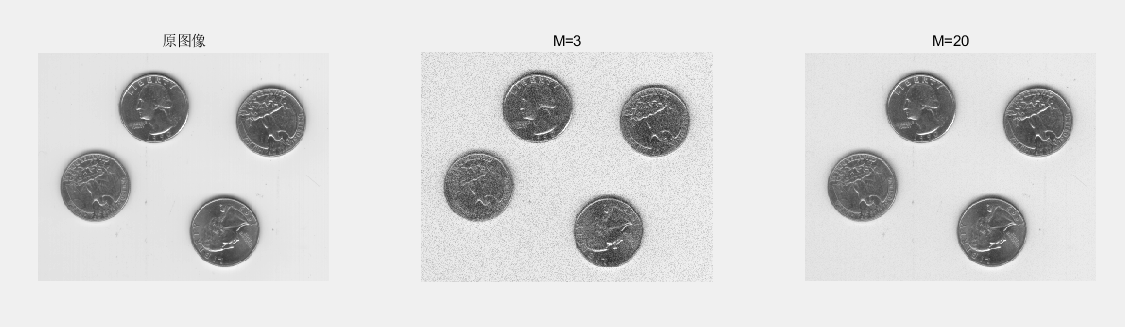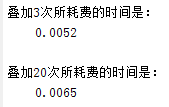2.图像的减法运算

图像的减法运算，常用于检测图像变化及物体运动。主要的应用在于检测同一场景下两幅图像之间的变化或是混合图像的分离。在MATLAB图像处理工具箱中提供了函数imsubtract()，可以将一幅图像从另一幅图像中减去（要求两幅图像的大小和类型都是相同的），或者从一幅图像中减去一个常数。注意：如果两幅图像都是uint8类型，减完之后结果是负数，那么将该负值四舍五入为0。

2.1 减去背景

下述代码是在imsubtract的帮助文档里看到的，对其中提取背景的方式存疑。

close all; clear all; clc;

I = imread('rice.png'); % 将图像读入工作区
background = imopen(I, strel('disk', 15));  % 估计背景
J = imsubtract(I, background); % 从图像中减去背景
figure;
subplot(131), imshow(I), title('原图');
subplot(132), imshow(background), title('背景');
subplot(133), imshow(J), title('原图-背景');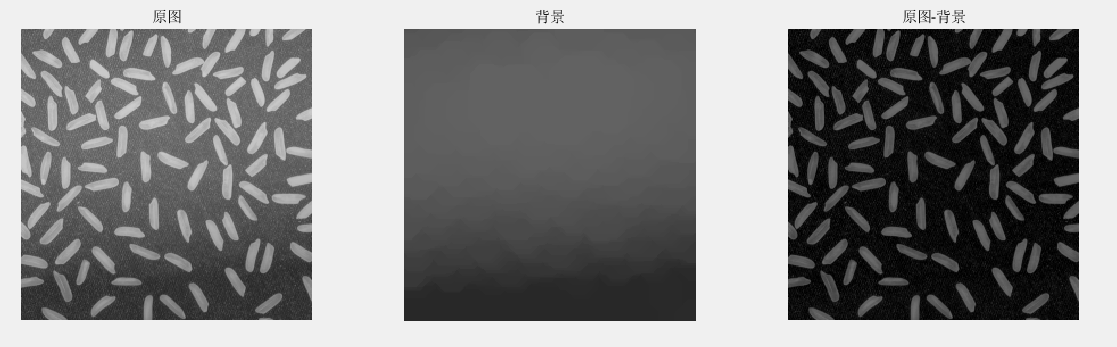2.2 利用图像减法运算实现混合图像分离

% 编写时间：2018年3月21日13:30:28
close all; clear all; clc;

A = im2double(imread('tire.tif'));  % 大小为205*232
B = im2double(imread('eight.tif')); % 大小为242*308
C = B;

[m, n] = size(A);

% 因两个图像的大小不相等，所以采用for循环语句进行逐点叠加，叠加的结果赋给C
for i = 1:m     % m = 205
for j = 1:n  % n = 232
C(i,j) = B(i, j) + A(i, j);
end
end
% imshow(C);
D = imabsdiff(C, B); % 求两图像对应像素点差的绝对值。为了避免产生负数
% D = imsubtract(C, B); % 在这里两幅图像都是double类型，相减之后的结果会产生负数
figure;
subplot(221), imshow(A);
subplot(222), imshow(B);
subplot(223), imshow(C);
subplot(224), imshow(D);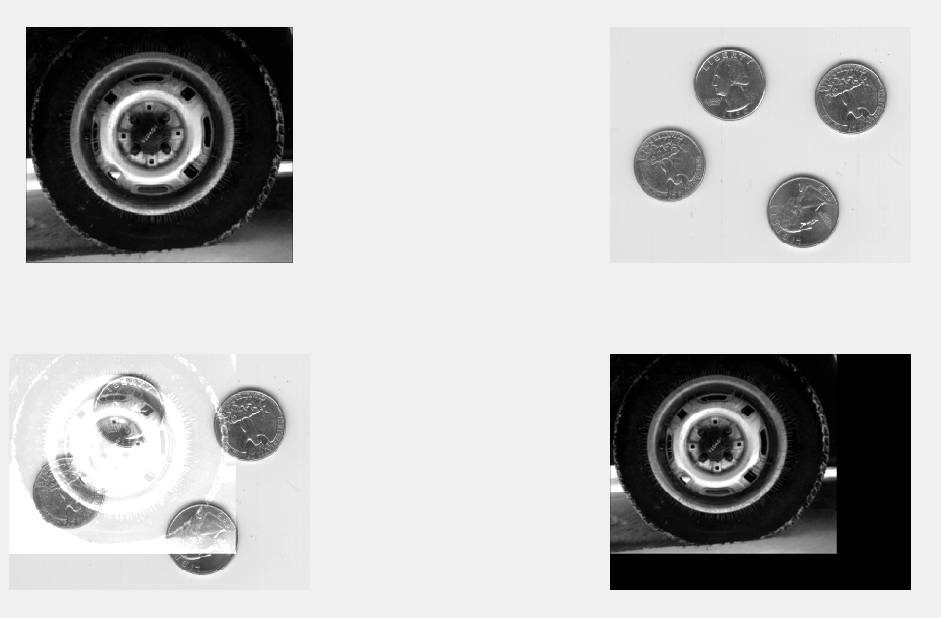3.图像的乘法运算

两幅图像进行乘法运算主要实现两个功能，一是可以实现掩膜操作，即屏蔽图像的某些部分；二是一幅图像乘以一个常数因子，如果该常数因子大于1，则图像亮度增强，如果该常数因子小于1，则图像亮度变暗。在MATLAB图像处理工具箱中提供的函数immultiply()可以实现两幅图像的乘法运算，该函数将两幅图像相应的像素值进行元素对元素的乘法运算（相当于MATLAB中矩阵的点乘），并将乘法的运算结果作为输出图像相应的像素值。

3.1 利用图像乘法运算实现图像局部显示

这里可能是没有选取好图像，所以效果不好。通过两幅图像相乘能够实现局部区域选择的作用，这可能对具体的图像是有要求的。

close all; clear all; clc;

A = im2double(imread('rice.png'));
B = im2double(imread('cameraman.tif'));
C = immultiply(A, B);
imshow(C);

3.2 利用图像乘法运算实现图像亮度的控制

close all; clear all; clc;

I = imread('house.jpg');
J1 = immultiply(I, 1.5);
J2 = immultiply(I, 0.5);

figure;
subplot(131), imshow(J1), title('变亮');
subplot(132), imshow(I), title('原图');
subplot(133), imshow(J2), title('变暗');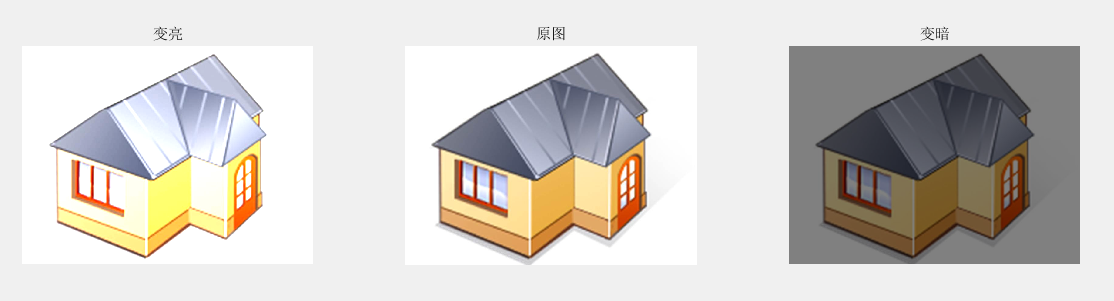4.图像的除法运算

图像的除法运算给出的是两幅图像相应像素值的变化比率，常用于校正成像设备的非线性影响。在MATLAB图像处理工具箱中提供了函数imdivide()实现两幅图像的除法，该函数对两幅输入图像的所有相应像素执行元素对元素的除法运算（即MATLAB中矩阵的点除操作），并将得到的结果作为输出图像的相应像素值。

4.1 利用函数imdivide()完成图像除法运算

close all; clear all; clc;

I = imread('office_1.jpg');
J = imread('office_2.jpg');

K1 = imdivide(J, I); % 两幅图像相除
K2 = imdivide(J, 0.5); % 一幅图像除以一个常数

figure;
subplot(221), imshow(I);
subplot(222), imshow(J);
subplot(223), imshow(K1);
subplot(224), imshow(K2);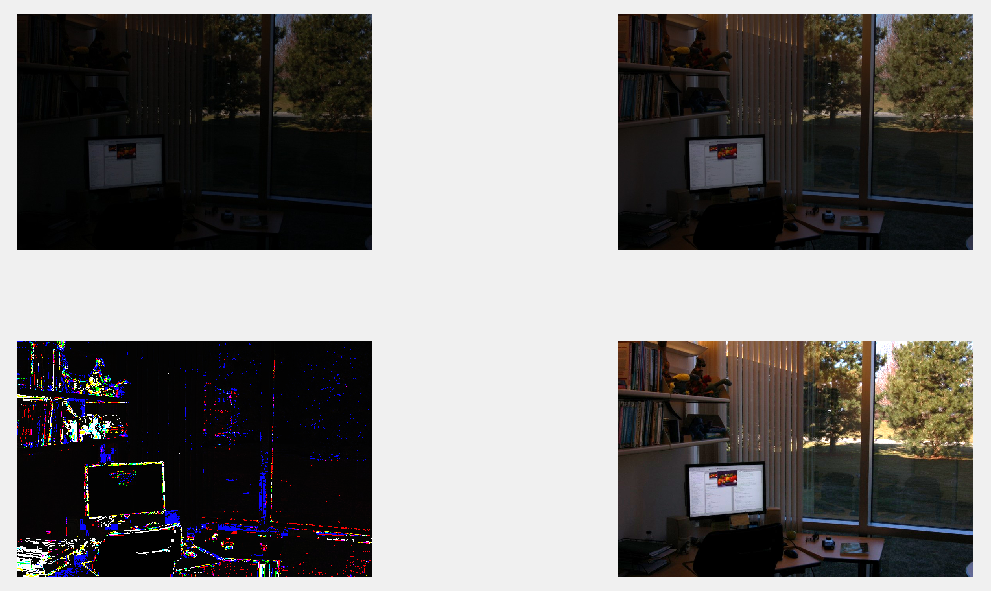由上图可知，K1返回图像I和图像J比率变换的差异图像，K2返回图像J和常数0.5相除的结果。两幅图像相除结果反映图像的线性度，图像与常数相除可实现图像的亮度调节。

5.其他的一些图像代数运算

5.1 图像求补函数imcomplement()

输入图像可以是二值图像、灰度图像、RGB图像。输出图像和输入图像具有同样的大小和数据类型。

close all; clear all; clc;

I = imread('rice.png');
J = imbinarize(I); % 二值化

I1 = imcomplement(I);  % 原始图像的补
J1 = imcomplement(J);  % 二值图像的补

figure;
subplot(221), imshow(I);
subplot(222), imshow(J);
subplot(223), imshow(I1);
subplot(224), imshow(J1);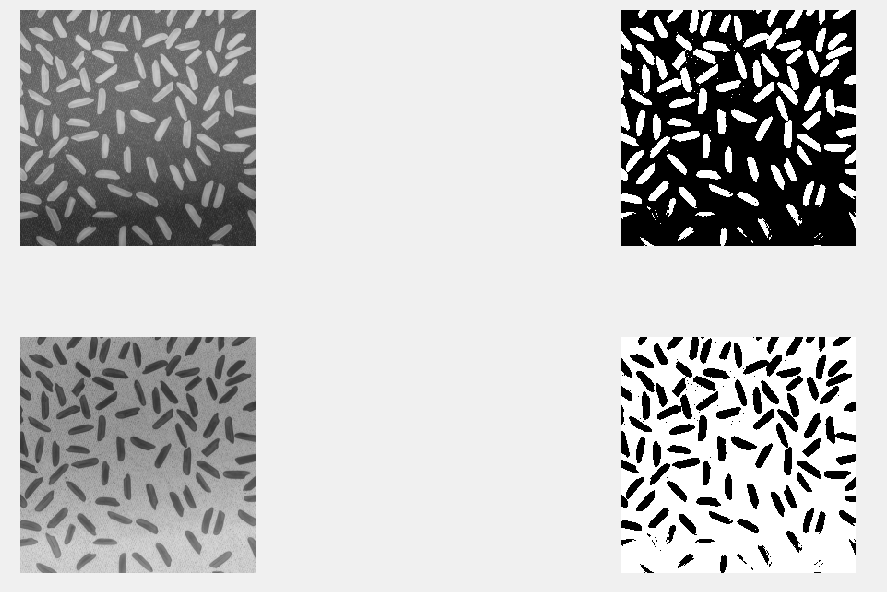5.2 绝对值差函数imsubtract()

该函数执行图像A和图像B对应位置的元素相减，并取绝对值。图像A和图像B要求具有相同大小和数据类型。

close all; clear all; clc;

I = imread('cameraman.tif'); % uint8

w = fspecial('prewitt');
J = filter2(w, I); % double

K = imabsdiff(double(I), J); % double

figure;
subplot(131), imshow(I);
subplot(132), imshow(J, []);
subplot(133), imshow(K, []);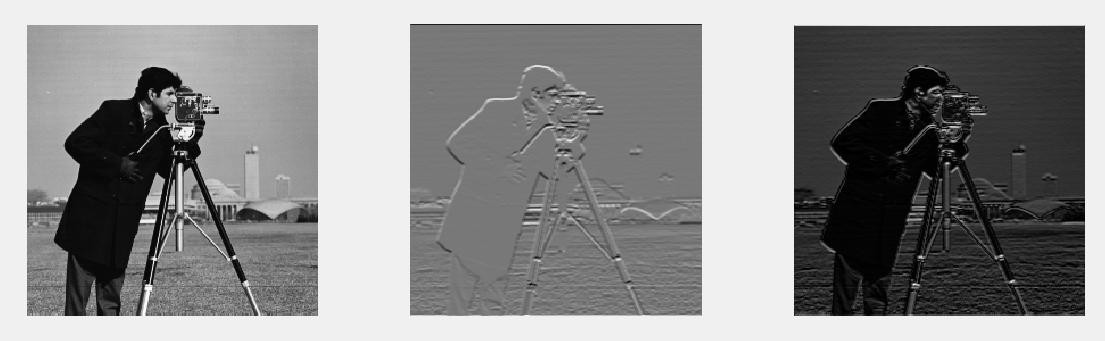5.3 图像运算的线性组合函数imlincomb()

函数形式是z=imlincomb(K1, A1, K2, A2, ... , Kn, An, K)，计算K1*A1+K2*A2+...+Kn*An+K的，结果返回给z。

close all; clear all; clc;

I = imread('cameraman.tif');
J = imread('rice.png');

K1 = imlincomb(1.0, I, 1.0, J);
K2 = imlincomb(1.0, I, -1.0, J, 'double'); % 这里的double选项是使K2转换为double类型
K3 = imlincomb(2, I);
K4 = imlincomb(0.5, I);

figure;
subplot(221), imshow(K1);
subplot(222), imshow(K2);
subplot(223), imshow(K3);
subplot(224), imshow(K4);三、图像逻辑运算

图像的逻辑运算主要针对二值图像，以像素对像素为基础进行的两幅或多幅图像间的操作。

1.实现图像的与、或、非、异或运算

tic;

close all; clear all; clc;

I = imread('rice.png');
J = imread('cameraman.tif');

I1 = im2bw(I); % 转二值化图像
J1 = im2bw(J);

K1 = I1 & J1;  % 与
K2 = I1 | J1;  % 或
K3 = ~ I1;     % 非
K4 = xor(I1, J1); % 异或

figure;
subplot(241), imshow(I), title('原图1');
subplot(242), imshow(J), title('原图2');
subplot(243), imshow(I1), title('二值图像1');
subplot(244), imshow(J1), title('二值图像2');
subplot(245), imshow(K1), title('与');
subplot(246), imshow(K2), title('或');
subplot(247), imshow(K3), title('非');
subplot(248), imshow(K4), title('异或');

toc;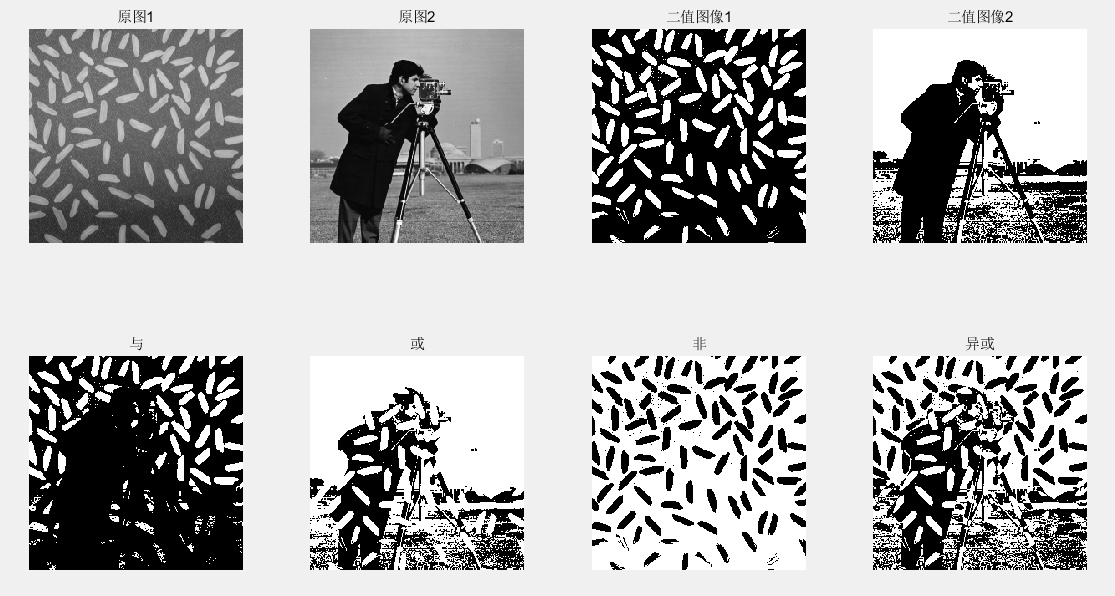2.实现图像的或非、与非运算

close all; clear all; clc;

I = rgb2gray(imread('lena.jpg'));
I1 = im2bw(I);

J = imread('rice.png');
J1 = im2bw(J);

K1 = ~(I1 | J1);  % 或非
K2 = ~(I1 & J1);  % 与非

figure;
subplot(231), imshow(I), title('lena');
subplot(232), imshow(J), title('rice');
subplot(233), imshow(I1), title('lena二值化');
subplot(234), imshow(J1), title('rice二值化');
subplot(235), imshow(K1), title('或非');
subplot(236), imshow(K2), title('与非');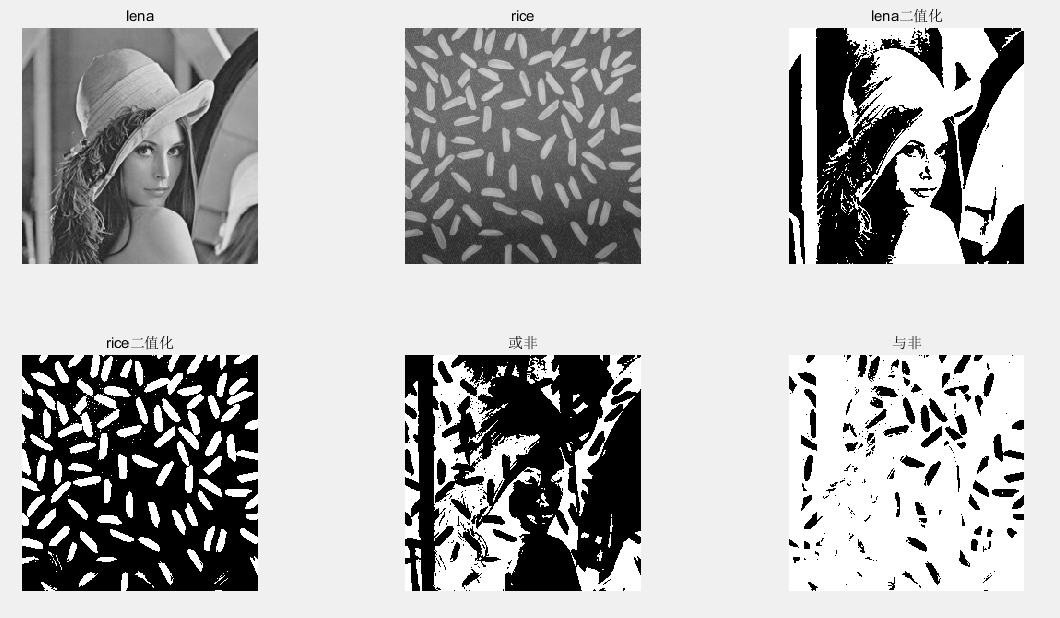四、图像的几何变换

图像的几何变换是将一幅图像中的坐标映射到另一幅图像中的新坐标位置，它不改变图像的像素值，只改变像素所在的几何位置，使原始图像按照需要发生位置、形状和大小的变化。这里主要包括图像的平移、镜像变换、转置、缩放等。

1.图像的平移

图像的平移是将一幅图像上的所有点都按照给定的偏移量在水平方向上沿x轴、在垂直方向上沿y轴移动。

1.1 实现图像的平移，不考虑平移后超出显示区域的像素点

首先写平移函数move_1，如下：

function J = move_1(I, a, b)
% MOVE_1 平移图像，不考虑超出显示区域的像素点
% 输入：
%     I -- 输入图像
%     a -- 沿y轴的偏移量
%     b -- 沿x轴的偏移量
% 输出：
%     J -- 输出图像

% created by gq

[M, N, G] = size(I);
I = im2double(I);

J = ones(M, N, G); % 这里的J是一个double类型的幺矩阵

for i = 1:M
for j = 1:N
if ((i+a)>=1 && (i+a)<=M && (j+b)>=1 && (j+b)<=N)
J(i+a, j+b, :) = I(i,j, :);
end
end
end

调用函数：

close all; clear all; clc;

I = imread('lena.jpg');

figure;
subplot(151), imshow(I), title('原图');
axis on;

J1 = move_1(I, 50, 50);
subplot(152), imshow(J1), title('向↓向→移动50个像素');
axis on;

J2 = move_1(I, -50, 50);
subplot(153), imshow(J2), title('向↑向→移动50个像素');
axis on;

J3 = move_1(I, 50, -50);
subplot(154), imshow(J3), title('向↓向←移动50个像素');
axis on;

J4 = move_1(I, -50, -50);
subplot(155), imshow(J4), title('向↑向←移动50个像素');
axis on; 

实验效果：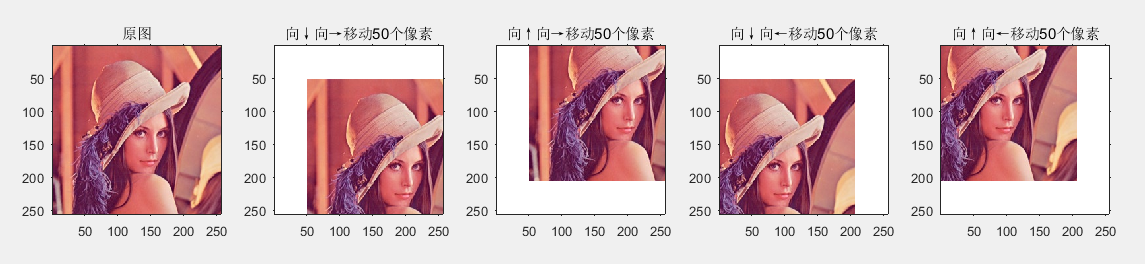1.2 实现图像的平移，考虑平移后超出显示区域的像素点

首先写平移函数move_2，如下：

function J = move_2(I, a, b)
% MOVE_2 平移图像，考虑超出显示区域的像素点
% 考虑平移以后图像溢出情况，采用扩大显示区域的方法
% 输入：
%     I -- 输入图像
%     a -- 沿y轴的偏移量
%     b -- 沿x轴的偏移量
% 输出：
%     J -- 输出图像

% created by gq

[M, N, G] = size(I);

I = im2double(I);

J = ones(M+abs(a), N+abs(b), G);

for i = 1:M
for j = 1:N
if (a<0 && b<0)  % 向上向左移动
J(i, j, :) = I(i, j, :);
elseif (a>0 && b<0)  % 向下向左移动
J(i+a, j, :) = I(i, j, :);
elseif (a>0 && b>0)  % 向下向右移动
J(i+a, j+b, :) = I(i, j, :);
else             % 向上向右移动
J(i, j+b, :) = I(i, j, :);
end
end
end

调用函数：

close all; clear all; clc;

I = imread('lena.jpg');

figure;
subplot(151), imshow(I), title('原图');
axis on;

J1 = move_2(I, 50, 50);
subplot(152), imshow(J1), title('向↓向→移动50个像素');
axis on;

J2 = move_2(I, -50, 50);
subplot(153), imshow(J2), title('向↑向→移动50个像素');
axis on;

J3 = move_2(I, 50, -50);
subplot(154), imshow(J3), title('向↓向←移动50个像素');
axis on;

J4 = move_2(I, -50, -50);
subplot(155), imshow(J4), title('向↑向←移动50个像素');
axis on; 

实验效果：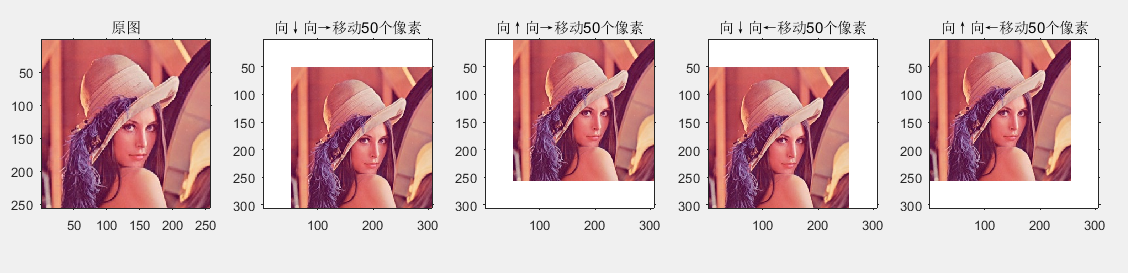2.图像的镜像

图像的镜像分为水平镜像和垂直镜像。水平镜像是指图像的左半部分和右半部分以图像竖直中轴线为中心轴进行对换。垂直镜像是指图像的上半部分和下半部分以图像水平中轴线为中心轴进行对换。

编写镜像函数mirror()，如下：

function dstImage = mirror(srcImage, direction)
% MIRROR 实现图像的镜像
% 输入：
%     srcImage -- 输入图像
%     direction -- 镜像的方向
%       当direction=1时，实现水平镜像
%       当direction=2时，实现垂直镜像
%       当direction=3是，实现水平垂直镜像
% 输出：
%     dstImage -- 输出图像

% created by gq

I = im2double(srcImage);

[M, N, G] = size(I); % 获取输入图像的大小

J = ones(M, N, G); % 建立一个与I大小相同的幺矩阵

if (direction == 1)  % 水平镜像
for i = 1:M
for j = 1:N
J(i, j, :) = I(i, N-j+1, :);
end
end
elseif (direction == 2)  % 垂直镜像
for i = 1:M
for j = 1:N
J(i, j, :) = I(M-i+1, j, :);
end
end
elseif (direction == 3) % 水平垂直镜像
for i = 1:M
for j = 1:N
J(i, j, :) = I(M-i+1, N-j+1, :);
end
end
else
error('参数输入不正确，第二个参数应取值为1,2,3');  % direction输入错误时报错
end

dstImage = J; 

调用函数：

close all; clear all; clc;

I = imread('lena.jpg');
J1 = mirror(I, 1); % 水平镜像
J2 = mirror(I, 2); % 垂直镜像
J3 = mirror(I, 3); % 水平垂直镜像

figure;
subplot(221), imshow(I), title('原始图像');
subplot(222), imshow(J1), title('水平镜像');
subplot(223), imshow(J2), title('垂直镜像');
subplot(224), imshow(J3), title('水平垂直镜像');

实验效果图：3.图像的缩放

MATLAB图像处理工具箱中提供了函数imresize()进行图像的缩放操作。调用格式如下（未考虑索引图像。函数的一些参数设置此处也未列出，参考imresize的帮助文档）：

B = imresize(A, m):该函数返回缩放后的图像B，A为要进行缩放操作的原始图像，可以是灰度图像、彩色图像或者二值图像；m为缩放的尺寸，当m的取值大于0小于1时，图像A被缩小，当m取值大于1时，图像A被放大。

B = imresize(A, [mrows, ncols]):该函数返回缩放后的图像B，A为要进行缩放操作的原始图像，可以是灰度图像、彩色图像或者二值图像；数组[mrows, ncols]说明缩放后B图像的行和列，mrows或者ncols取值为NaN，则函数会按照输入图像A的从横比生成ncols或者mrows的数。

% 图像缩放的代码

close all; clear all; clc;

I = imread('lena.jpg');

J1 = imresize(I, 0.5); % 缩放0.5倍
J2 = imresize(I, 1.5); % 缩放1.5倍

J3 = imresize(I, [64, 40]); % 缩放之后的图像J3大小为64*40
J4 = imresize(I, [64, NaN]); % 缩放之后的图像J3行为64，列按I的从横比对应生成

J5 = imresize(I, 1.5, 'bilinear'); % 采用双线性插值，此处还可选择最近邻插值'nearest'和双三次插值'bicubic'
J6 = imresize(I, 1.5, 'triangle'); % 插值的核函数为triangle（三角型核函数），此处未说明插值方法，默认为nearest

J7 = imresize(I, 'scale', 0.5); % 缩放比例设置为0.5，与imresize(I, 0.5)相同

figure;
subplot(241), imshow(I);
subplot(242), imshow(J1);
subplot(243), imshow(J2);
subplot(244), imshow(J3);
subplot(245), imshow(J4);
subplot(246), imshow(J5);
subplot(247), imshow(J6);
subplot(248), imshow(J7);由上图可以看见：缩小后的图像会丢失一部分原图像的信息，所以显示出现模糊化；放大后的图像，增加了原图像的信息，显示的更加清晰。指定行列的图像缩放中，建议采用原图像的纵横比，这样缩放后能更好的地保持图像信息。

4.图像的转置

图像的转置即图像的行列坐标互换。需要注意的是，进行图像转置后，图像的大小会发生变化。在MATLAB中没有提供实现图像转置的函数，直接运用MATLAB指令编程即可实现图像的转置操作。

编写图像转置函数transp()，如下：

function dstImage = transp(srcImage)
% TRANSP 实现图像的转置
% 输入：
%     srcImage -- 输入图像
% 输出：
%     dstImage -- 经过转置之后的图像

% created by gq
% 2018年4月26日22:52:53

[M, N, G] = size(srcImage); % 获得输入图像srcImage的大小
srcImage = im2double(srcImage); % 将图像的数据类型转换为双精度类型
dstImage = ones(N, M, G); % 注意这里图像的大小发生了变化

for i = 1:N
for j = 1:M
dstImage(i, j, :) = srcImage(j, i, :);
end
end

调用函数：

close all; clear all; clc;

I1 = imread('C:\Users\Administrator\Desktop\yasuo.jpg');
J1 = transp(I1);

I2 = imread('lena.jpg');
J2 = transp(I2);

figure;
subplot(221), imshow(I1);
subplot(222), imshow(J1);
subplot(223), imshow(I2);
subplot(224), imshow(J2); 

实验效果：5.图像的旋转

图像的旋转属于图像的位置变换，通常以图像的中心为原点，将图像上的所有像素都旋转一个相同的角度。旋转后，图像的大小一般会发生变化。在MATLAB图像处理工具箱中提供了函数imrotate()进行图像的旋转操作，其调用格式如下：

B=imrotate(A, angle)：该函数是将图像A按照角度angle以图像中心为原点进行旋转。angle取值大于0，按照逆时针方向旋转；angle取值小于0，按照顺时针方向旋转。该函数利用'nearest'方法进行邻域插值，能够生成完整的旋转图像B.

B=imrotate(A, angle, method)：该函数是将图像A按照角度angle以图像中心为原点进行旋转。旋转时采用method的方法进行插值，method取值为'nearest'（默认值）最近邻插值，'bilinear'双线性插值，'bicubic'双三次插值。

B=imrotate(A, angle, method, bbox)：该函数是将图像A按照角度angle以图像中心为原点进行旋转。采用method的方法进行插值，bbox说明返回图像的大小，其取值可以是'crop'或者'loose'，其中'crop'表示输出图像大小和输入图像大小相等，对旋转后的图像进行裁剪，'loose'（默认值）表示使输出的图像足够大包括完整的旋转图像。

代码如下：

close all; clear all; clc;

I = imread('office_2.jpg');

J1 = imrotate(I, 30); % 逆时针旋转30°
J2 = imrotate(I, -30); % 顺时针旋转30°

J3 = imrotate(I, 30, 'bicubic', 'crop'); % 逆时针旋转30°，bicubic插值，输出图像和输入图像的大小相等
J4 = imrotate(I, 30, 'bicubic', 'loose');% 逆时针旋转30°，bicubic插值，输出图像足够大能够包括完整的旋转图像

figure;
subplot(151), imshow(I);
subplot(152), imshow(J1);
subplot(153), imshow(J2);
subplot(154), imshow(J3);
subplot(155), imshow(J4); 

实现效果图：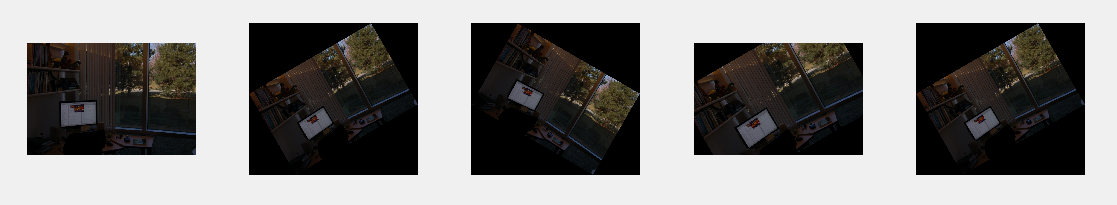6.图像的剪切

在MATLAB图像处理工具箱中提供了函数imcrop()进行图像的剪切操作，其具体的调用格式如下：

I2=imcrop(I, rect):该函数是按照四元素数组rect剪切图像I，rect的具体形式为[xmin, ymin, width, height]说明剪切矩形区域的大小。

[I2, rect]=imcrop(I):该函数执行后首先显示原图像，然后利用鼠标选择剪切区域，并将剪切区域图像返回给I2，将剪切区域的范围大小返回给rect。

[X, Y, I2, rect]=imcrop(I):该函数执行后首先显示原图像，然后利用鼠标选择剪切区域，返回当前剪切区域图像的像素点x和y坐标给X和Y，将剪切区域图像返回给I2，将剪切区域的范围大小返回给rect。

6.1 利用函数imcrop()，通过指令方式实现图像的剪切操作

close all; clear all; clc;

I = imread('lena.jpg');
rect = [90, 90, 100, 112];
J = imcrop(I, rect);

figure;
subplot(121), imshow(I);
rectangle('Position', rect, 'LineWidth', 2, 'EdgeColor', 'b'); % 为了突出剪切区域
subplot(122), imshow(J);6.2  利用函数imcrop()，通过鼠标操作实现图像的剪切操作

close all; clear all; clc;

I = imread('lena.jpg');

[J, rect] = imcrop(I);

figure;
subplot(121), imshow(I);
rectangle('Position', rect, 'LineWidth', 2, 'EdgeColor', 'b');
subplot(122), imshow(J); 

程序执行后，首先显示lena图像，当鼠标移至图像区域后，光标变成“+”的形状，按住鼠标左键选择剪切区域，再在选择的剪切区域内右击，弹出剪切菜单，选择crop Image选项，即可。如下图：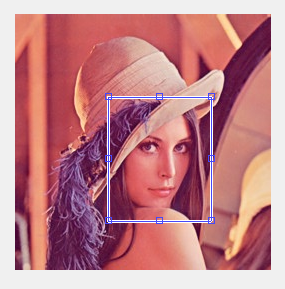7.图像的空间变换

在MATLAB图像处理工具箱中提供了一个专门的函数imtransform()，用户可以定义参数实现多种类型的空间变换，包括仿射变换（如：平移、缩放、旋转、剪切）、投影变换等。函数imtransform()的调用格式如下：

B=imtransform(A, TFORM):该函数中A是待变换的图像矩阵；TFORM表示执行空间变换的所有参数的结构体，它是通过函数maketform()返回的，B为按照TFORM参数变换后的图像矩阵。

B=imtransform(A, TFORM, INTERP):该函数中参数INTERP说明图像空间变换时的插值类型，它的取值类型可以是'nearest', 'bilinear', 'bicubic'，默认为'bilinear', 其他参数与B=imtransform(A, TFORM)调用方式相同。

[B, XDATA, YDATA]=imtransform(..., param1, val1, param2, val2, ...):该函数中用户可以自己指定空间变换的各个参数，param1和param2表示参数名称，val1和val2为对应参数的取值，（具体参数表doc imtransform），返回结果中除了变换后的图像B，同时还返回图像B在X-Y空间中的位置XDATA和YDATA。XDATA返回图像B中从第一列到最后一列在x轴的坐标，YDATA返回图像B从第一行到最后一行在y轴的坐标。

在MATLAB中利用函数imtransform()实现图像的空间变换时，都需要先定义空间变换的参数。对于空间变换参数的定义，MATLAB也提供了相应的函数maketform()，它的作用是创建进行空间变换的参数结构体，调用方式如下：

T=maketform('affine', A): 该函数返回一个N维的仿射性变换参数结构体T，输入参数A是一个(N+1)*(N+1)或者(N+1)*N的矩阵。如果A是(N+1)*(N+1)的矩阵，A的最后一列为[zeros(N,1); 1]。

T=maketform('affine', U, X): 该函数返回的是一个二维仿射性变换参数结构体T，输入U的每一行映射X的每一行，U和X都是3*2的矩阵，其中U是输入三角形三个角的空间坐标矩阵，X是映射得到的三角形三个角的空间坐标矩阵。

T=maketform('projective', A): 该函数返回一个N维投影变换结构体参数T，A是一个(N+1)*(N+1)的非奇异矩阵，A(N+1，N+1)不能为0。

T=maketform('projective', U, X): 该函数返回一个二维投影变换结构体参数T，输入U的每一行映射X的每一行，U和X都是4*2的矩阵，其中U是输入四边形顶角的空间坐标变换，X是映射得到的四边形四个顶点的空间坐标矩阵。

T=maketform('custom', NDIMS_IN, NDIMS_OUT, FORWARD_FCN, INVERSE_FCN, TDATA): 该函数返回一个用户自定义变换的参数结构体T。NDIMS_IN, NDIMS_OUT表示输入和输出的维数，FORWARD_FCN, INVERSE_FCN表示正向变换函数和逆向变换函数的句柄。

用户结合使用函数maketform()和函数imtransform()，就可以灵活地实现图像的线性变换，而变换的结果和变换参数结构体密切相关。

7.1 利用函数imtransform()，实现图像的平移、缩放、旋转和剪切

close all; clear all; clc;

I = imread('lena.jpg');

figure;
subplot(231), imshow(I), axis on, title('原图像');

% 创建旋转参数结构体
T1 = maketform('affine', [cosd(30), -sind(30), 0; sind(30), cosd(30), 0; 0, 0, 1]);
% 实现图像旋转
J1 = imtransform(I, T1);
subplot(232), imshow(J1), axis on, title('图像旋转');

% 创建缩放参数结构体
T2 = maketform('affine', [2, 0, 0; 0, 1, 0; 0, 0, 1]);
% 实现图像缩放
J2 = imtransform(I, T2);
subplot(233), imshow(J2), axis on, title('图像缩放');

% 创建平移参数结构体
xform = [1, 0, 55; 0, 1, 115; 0, 0, 1]';
T3 = maketform('affine', xform);
% 实现图像平移
J3 = imtransform(I, T3, 'XData', [1, (size(I,2)+xform(3,1))], 'YData', ...
[1, (size(I,1)+xform(3,2))], 'FillValues', 0);
subplot(234), imshow(J3), axis on, title('图像平移');

% 创建图像整体切变得参数结构体
T4 = maketform('affine', [1, 4, 0; 2, 1, 0; 0, 0, 1]');
% 实现图像的切变
J4 = imtransform(I, T4, 'FillValues', 255);
subplot(235), imshow(J4), axis on, title('图像切变');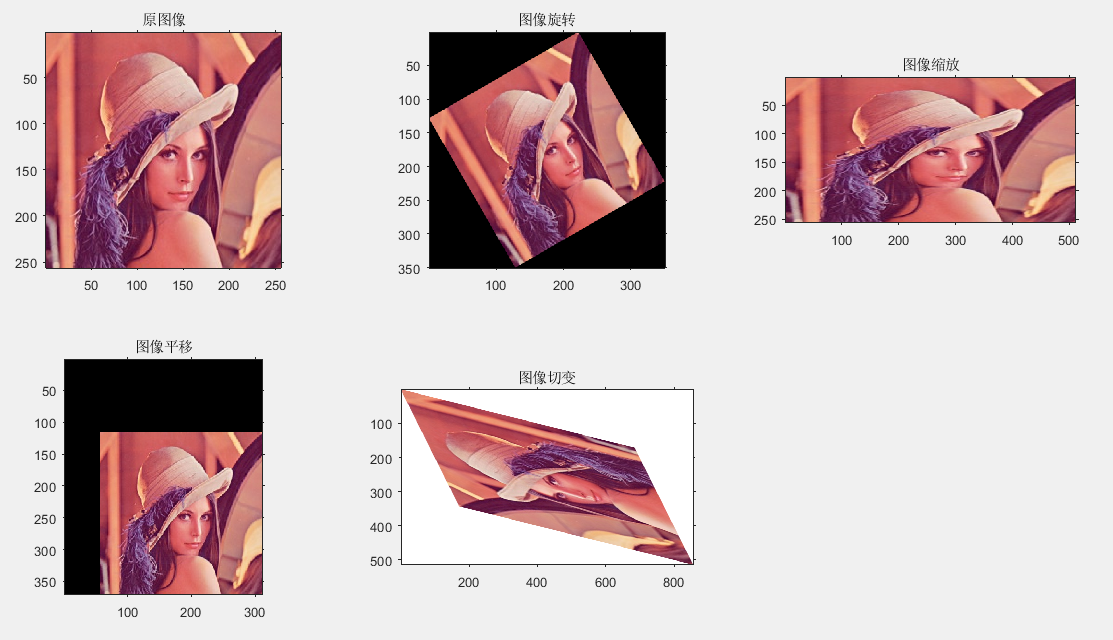五、图像的邻域和块操作

1.图像的邻域操作

图像的邻域操作是指输出图像的像素点取值决定于输入图像的某个像素点及其邻域内的像素，通常像素点的邻域是一个远小于图像自身大小、形状规则的像素块，如2*2正方形、2*3矩形、或近似于圆形的多边形。邻域操作根据邻域的类型又可分为滑动邻域操作和分离邻域操作。

1.1 通用滑动邻域操作函数nlfilter()

B=nlfilter(A, [m, n], fun)：该函数返回图像B，它是输入灰度图像A，按照大小为m*n滑动邻域，利用运算函数fun处理之后的结果。其中fun可以是向量平均值mean，矩阵的平均值mean2，向量方差std，矩阵方差std2，向量最小值min，最大值max，方差var，也可以是用inline自定义函数。

close all; clear all; clc;

A = im2double(imread('cameraman.tif'));
figure;
subplot(221), imshow(A);

B1 = nlfilter(A, [4, 4], 'std2'); % 对图像A按4*4滑动邻域计算方差
subplot(222), imshow(B1);

fun = @(x) max(x(:));

B2 = nlfilter(A, [3, 3], fun);
subplot(223), imshow(B2);

B3 = nlfilter(A, [6, 6], fun);
subplot(224), imshow(B3);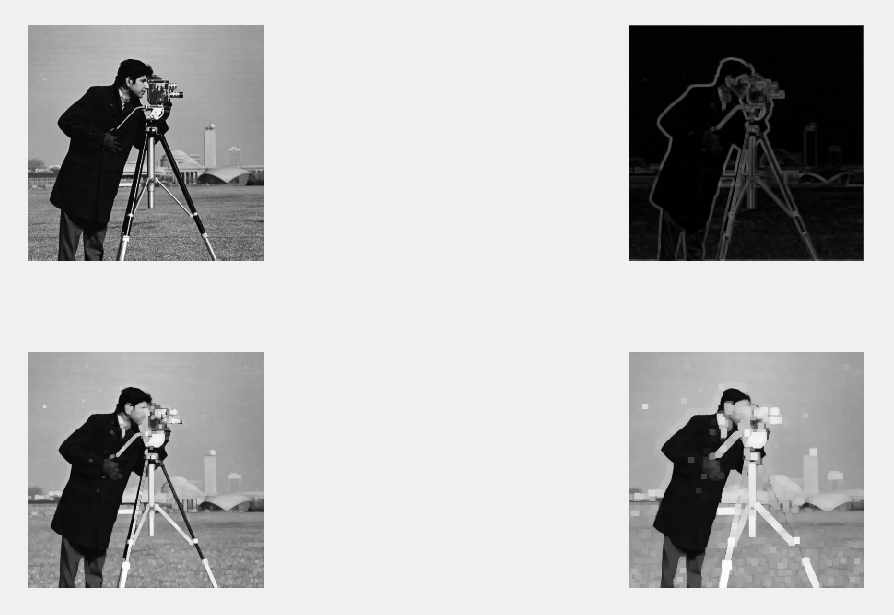1.2 列方向邻域操作函数colfilt()

B=colfilt(A, [m, n], block_type, fun)：该函数将输入图像A，按照大小为m*n块重新组合成一个临时列矩阵，利用函数fun对这个临时列矩阵进行处理，如果需要填充则用0对图像A进行填充。其中block_type是字符串，可以取'distinct'或'sliding'，取'distinct'按照分离邻域方式重新组合临时矩阵，处理后返回的矩阵大小必须和临时矩阵相同；取'sliding'表示按照滑动邻域方式重新组合临时矩阵，处理后返回一个行向量，向量中包含临时矩阵中每一行一个单一值。

close all; clear all; clc;

I = im2double(imread('tire.tif'));
figure;
subplot(221), imshow(I);

fun = @(x) min(x);
I2 = colfilt(I, [4, 4], 'sliding', fun);
subplot(222), imshow(I2);

m = 2;
n = 2;
fun = @(x) ones(m*n, 1) * min(x);
I3 = colfilt(I, [m, n], 'distinct', fun);
subplot(223), imshow(I3);

m = 4;
n = 4;
fun = @(x) ones(m*n, 1) * min(x);
I4 = colfilt(I, [m, n], 'distinct', fun);
subplot(224), imshow(I4); 

1.3 分离邻域操作函数blockproc()

B=blockproc(A, [m,n], fun)：该函数对输入图像A，采用大小为m*n的分离块，利用函数fun处理，处理后的结果连接成输出图像B。其中fun是函数句柄，该函数输入为结构体，返回矩阵，向量或变量。

close all; clear all; clc;

I = imread('peppers.png');

fun = @(block_struct) imrotate(block_struct.data, 30);
I1 = blockproc(I, [64, 64], fun);

fun = @(block_struct) std2(block_struct.data);
I2 = blockproc(I, [32, 32], fun);

fun = @(block_struct) block_struct.data(:, :, [3, 1, 2]);
blockproc(I, [100, 100], fun, 'Destination', 'brg_peppers.tif');

figure;
subplot(221), imshow(I);
subplot(222), imshow(I1);
subplot(223), imshow(I2, []);
subplot(224), imshow('brg_peppers.tif');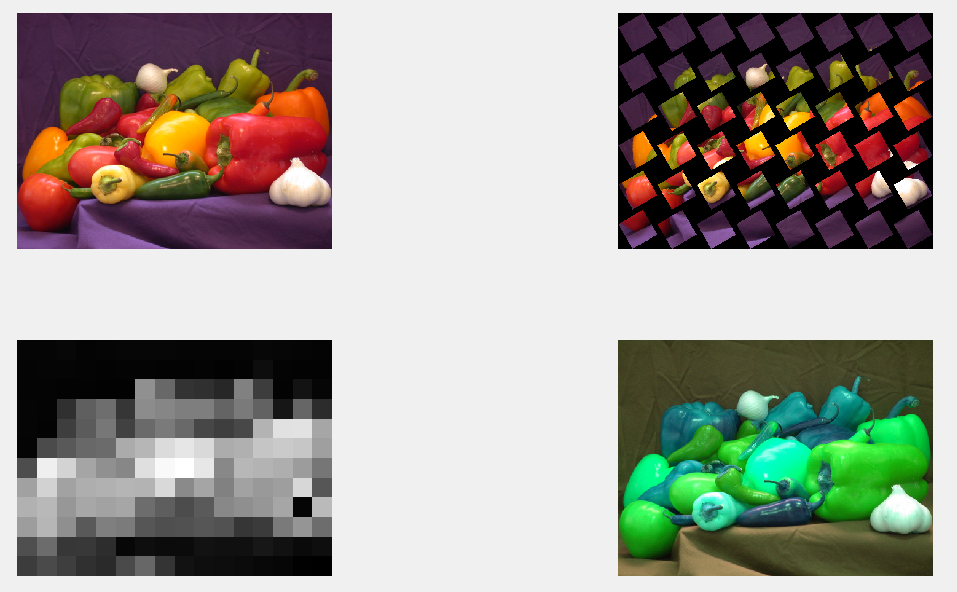展开全文• 通过GUI用户界面实现了数字图像的读取、存储、剪切、反色、加噪、滤波去噪、边沿检测、旋转、撤销和还原等功能。完成了一个功能齐全，运行稳定的数字图像处理系统的设计。（包含可执行代码及编译文件）
• 如何利用MATLAB提供GUI界面实现...通过GUI用户界面实现了数字图像的读取、存储、剪切、反色、加噪、滤波去噪、边沿检测、旋转、撤销和还原等功能。完成了一个功能齐全，运行稳定的数字图像处理系统的设计。（附代码）gui
• 继上次介绍了三种基本的图像数字水印算法以及代码后，其中大部分实验者都需要进行水印的抗攻击实验来测量算法的性能。上次文中也提到后期会介绍图像攻击算法，今天就介绍几个简单...剪切图像'); disp('4-->旋转攻...计算机视觉
• 流程包括，读取宿主图像和水印图像，嵌入，多种方法的攻击（剪切，加噪，旋转等），提取，最后利用psnr峰值信噪比进行评价不同攻击下，鲁棒性的好坏。二、GUI界面1、主界面2、dct子界面 3、dwt子界面4、其中dwt方法....
• 流程包括，读取宿主图像和水印图像，嵌入，多种方法的攻击（剪切，加噪，旋转等），提取，最后利用psnr峰值信噪比进行评价不同攻击下，鲁棒性的好坏。二、GUI界面设计及预期效果图1、主界面主界面GUI 2、dct子界面...
• 1、能对图像进行平移、旋转、缩放、剪切、投影、仿射变换以及各种复合变换； 2、能将各种变换后结果保存为图像文件； 3、各种变换可以采用按钮或者菜单的方式进行； 4、变换时的用户可以自行设置简单的变换参数。 二...GUI
• 一、课题介绍 本课题为基于MATLAB的小波变换dwt和离散余弦dct的...流程包括，读取宿主图像和水印图像，嵌入，多种方法的攻击（剪切，加噪，旋转等），提取，最后利用psnr峰值信噪比进行评价不同攻击下，鲁棒性的好坏。
• 基于matlab的数字图像水印系统，基于DCT算法实现水印的嵌入和提取，能进行不同类型的攻击——不同角度旋转、不同方差加噪、不同位置剪切和不同程度压缩。同时在攻击时返回PSNR、模糊系数、质量指标Q用以判断鲁棒性
• 基于matlab的数字图像水印系统，基于DWT算法一级小波实现水印的嵌入和提取，能进行不同类型的攻击——不同角度旋转、不同方差加噪、不同位置剪切和不同程度压缩。同时在攻击时返回PSNR、模糊系数、质量指标Q用以判断...
• 一、课题介绍 ...流程包括，读取宿主图像和水印图像，嵌入，多种方法的攻击（剪切，加噪，旋转等），提取，最后利用psnr峰值信噪比进行评价不同攻击下，鲁棒性的好坏。 二、GUI界面 1、主界面 ![主界面...计算机视觉 数字信号处理
• 源码： ...一、课题介绍 本课题为基于MATLAB的小波变换dwt和离散余弦dct的多方法对比数字水印系统。...流程包括，读取宿主图像和水印图像，嵌入，多种方法的攻击（剪切，加噪，旋转等），提取，最后利...计算机视觉 自然语言处理 深度学习 机器学习
• 图像的仿射变换是指在空间直角坐标系中将一个二维坐标转换到另外一个二维坐标，仿射变换是一种线性变换，所以可以用矩阵的运算来表过仿射变换，仿射变换主要用来实现平移、缩放、翻转、旋转剪切等相关几何操作。...
• 1、能对图像进行平移、旋转、缩放、剪切、投影、仿射变换以及各种复合变换（复合变换指融合前面两种及以上的变换，比如既有平移又有旋转）； 2、能将各种变换后结果保存为图像文件； 3、各种变换可以采用按钮或者...
• 将早前开发的一些小软件在sourceforge开源，...数字图像攻击系统：数字图像常用的攻击，包括缩放、滤波、剪切、旋转等。 小波分析系统：小波在图像处理内的使用，包括：数字水印、图像压缩等。 希望大家各取所需...
• JX007基于MATLAB的电力系统仿真 JX008基于ProE轴类零件工艺设计 JX009基于特征的装配模型化和顺序生成 JX010基于约束的生产组设计和装配模拟的研究与开发 JX011集成式舵轮杠杆开启穴播器的设计 JX012零件设计 JX013...
• 流程包括，读取宿主图像和水印图像，嵌入，多种方法的攻击（剪切，加噪，旋转等），提取，最后利用psnr峰值信噪比进行评价不同攻击下，鲁棒性的好坏。二、GUI界面1、主界面2、dct子界面 3、dwt子界面4、其中dwt方法....
• 流程包括，读取宿主图像和水印图像，嵌入，多种方法的攻击（剪切，加噪，旋转等），提取，最后利用psnr峰值信噪比进行评价不同攻击下，鲁棒性的好坏。二、GUI界面设计及预期效果图1、主界面主界面GUI 2、dct子界面...# matlab图像剪切、旋转matlab 订阅